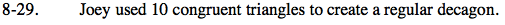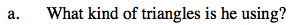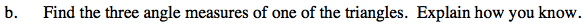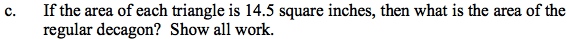Home > GC > Chapter 8 > Lesson 8.1.3 > Problem8-29

8-29.
1. Joey used 10 congruent triangles to create a regular decagon. Homework Help ✎

1. What kind of triangles is he using?

2. Find the three angle measures of one of the triangles. Explain how you know.

3. If the area of each triangle is 14.5 square inches, then what is the area of the regular decagon? Show all work.If the triangles are congruent, what does that mean for the ''radius'' lengths?
The radius goes from the center of the figure to a vertex on the figure.If there are 10 triangles, what is the measure of each central angle?

If this is an isosceles triangle, what is the measure of the other two base angles?The total area is equal to the area of all 10 of these triangles.